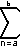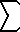Dave's Math Tables: Series Properties(Math | Calculus | Expansions | Series | Properties)

Semi-Formal Definition of a "Series":
A seriesan is the indicated sum of all values of an when n is set to each integer from a to b inclusive; namely, the indicated sum of the values aa + aa+1 + aa+2 + ... + ab-1 + ab.

Definition of the "Sum of the Series":
The "sum of the series" is the actual result when all the terms of the series are summed.

Note the difference: "1 + 2 + 3" is an example of a "series," but "6" is the actual "sum of the series."

Algebraic Definition:an = aa + aa+1 + aa+2 + ... + ab-1 + ab

Summation Arithmetic:c an = can (constant c)an +bn =an + bnan -bn =an - bn

Summation Identities on the Bounds:
 ban n=a c +an   n=b+1 c =an   n = a

 ban n=a b-c =an+c    n=a-c
 ban n=a b/c =anc    n=a/c
|
(similar relations exist for subtraction and division as generalized below for any operation g)
|

 ban n=a g(b) =ag -1(c)    n=g(a)